# 1 Introduction

This vignette will demonstrate a full single-cell lineage analysis workflow, with particular emphasis on the processes of lineage reconstruction and pseudotime inference. We will make use of the slingshot package proposed in (Street et al. 2017) and show how it may be applied in a broad range of settings.

The goal of slingshot is to use clusters of cells to uncover global structure and convert this structure into smooth lineages represented by one-dimensional variables, called “pseudotime.” We provide tools for learning cluster relationships in an unsupervised or semi-supervised manner and constructing smooth curves representing each lineage, along with visualization methods for each step.

## 1.1 Overview

The minimal input to slingshot is a matrix representing the cells in a reduced-dimensional space and a vector of cluster labels. With these two inputs, we then:

• Identify the global lineage structure by constructing an minimum spanning tree (MST) on the clusters, with the getLineages function.
• Construct smooth lineages and infer pseudotime variables by fitting simultaneous principal curves with the getCurves function.
• Assess the output of each step with built-in visualization tools.

## 1.2 Datasets

We will work with two simulated datasets in this vignette. The first (referred to as the “single-trajectory” dataset) is generated below and designed to represent a single lineage in which one third of the genes are associated with the transition. This dataset will be contained in a SingleCellExperiment object (Lun and Risso 2017) and will be used to demonstrate a full “start-to-finish” workflow.

# generate synthetic count data representing a single lineage
means <- rbind(
# non-DE genes
matrix(rep(rep(c(0.1,0.5,1,2,3), each = 300),100),
ncol = 300, byrow = TRUE),
# early deactivation
matrix(rep(exp(atan( ((300:1)-200)/50 )),50), ncol = 300, byrow = TRUE),
# late deactivation
matrix(rep(exp(atan( ((300:1)-100)/50 )),50), ncol = 300, byrow = TRUE),
# early activation
matrix(rep(exp(atan( ((1:300)-100)/50 )),50), ncol = 300, byrow = TRUE),
# late activation
matrix(rep(exp(atan( ((1:300)-200)/50 )),50), ncol = 300, byrow = TRUE),
# transient
matrix(rep(exp(atan( c((1:100)/33, rep(3,100), (100:1)/33) )),50),
ncol = 300, byrow = TRUE)
)
counts <- apply(means,2,function(cell_means){
total <- rnbinom(1, mu = 7500, size = 4)
rmultinom(1, total, cell_means)
})
rownames(counts) <- paste0('G',1:750)
colnames(counts) <- paste0('c',1:300)
sce <- SingleCellExperiment(assays = List(counts = counts))

The second dataset (the “bifurcating” dataset) consists of a matrix of coordinates (as if obtained by PCA, ICA, diffusion maps, etc.) along with cluster labels generated by $$k$$-means clustering. This dataset represents a bifurcating trajectory and it will allow us to demonstrate some of the additional functionality offered by slingshot.

library(slingshot, quietly = FALSE)
data("slingshotExample")
rd <- slingshotExample$rd cl <- slingshotExample$cl

dim(rd) # data representing cells in a reduced dimensional space
##  140   2
length(cl) # vector of cluster labels
##  140

# 2 Upstream Analysis

## 2.1 Gene Filtering

To begin our analysis of the single lineage dataset, we need to reduce the dimensionality of our data and filtering out uninformative genes is a typical first step. This will greatly improve the speed of downstream analyses, while keeping the loss of information to a minimum.

For the gene filtering step, we retained any genes robustly expressed in at least enough cells to constitute a cluster, making them potentially interesting cell-type marker genes. We set this minimum cluster size to 10 cells and define a gene as being “robustly expressed” if it has a simulated count of at least 3 reads.

# filter genes down to potential cell-type markers
# at least M (15) reads in at least N (15) cells
geneFilter <- apply(assays(sce)$counts,1,function(x){ sum(x >= 3) >= 10 }) sce <- sce[geneFilter, ] ## 2.2 Normalization Another important early step in most RNA-Seq analysis pipelines is the choice of normalization method. This allows us to remove unwanted technical or biological artifacts from the data, such as batch, sequencing depth, cell cycle effects, etc. In practice, it is valuable to compare a variety of normalization techniques and compare them along different evaluation criteria, for which we recommend the scone package (Cole and Risso 2018). We also note that the order of these steps may change depending on the choice of method. ZINB-WaVE (Risso et al. 2018) performs dimensionality reduction while accounting for technical variables and MNN (Haghverdi et al. 2018) corrects for batch effects after dimensionality reduction. Since we are working with simulated data, we do not need to worry about batch effects or other potential confounders. Hence, we will proceed with full quantile normalization, a well-established method which forces each cell to have the same distribution of expression values. FQnorm <- function(counts){ rk <- apply(counts,2,rank,ties.method='min') counts.sort <- apply(counts,2,sort) refdist <- apply(counts.sort,1,median) norm <- apply(rk,2,function(r){ refdist[r] }) rownames(norm) <- rownames(counts) return(norm) } assays(sce)$norm <- FQnorm(assays(sce)$counts) ## 2.3 Dimensionality Reduction The fundamental assumption of slingshot is that cells which are transcriptionally similar will be close to each other in some reduced-dimensional space. Since we use Euclidean distances in constructing lineages and measuring pseudotime, it is important to have a low-dimensional representation of the data. There are many methods available for this task and we will intentionally avoid the issue of determining which is the “best” method, as this likely depends on the type of data, method of collection, upstream computational choices, and many other factors. We will demonstrate two methods of dimensionality reduction: principal components analysis (PCA) and uniform manifold approximation and projection (UMAP, via the uwot package). When performing PCA, we do not scale the genes by their variance because we do not believe that all genes are equally informative. We want to find signal in the robustly expressed, highly variable genes, not dampen this signal by forcing equal variance across genes. When plotting, we make sure to set the aspect ratio, so as not to distort the perceived distances. pca <- prcomp(t(log1p(assays(sce)$norm)), scale. = FALSE)
rd1 <- pca$x[,1:2] plot(rd1, col = rgb(0,0,0,.5), pch=16, asp = 1)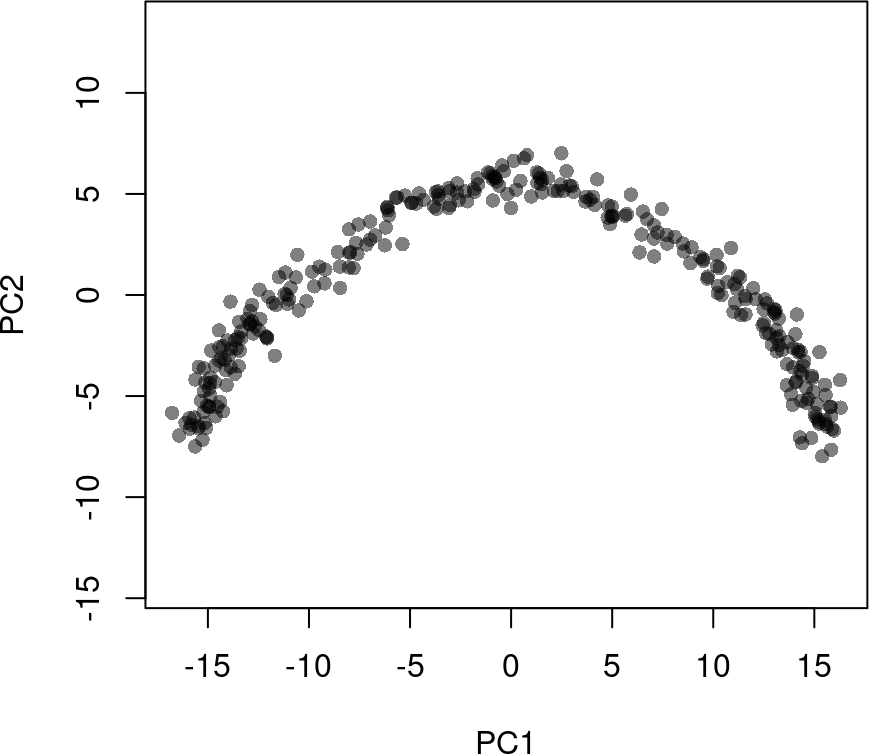library(uwot) ## Loading required package: Matrix ## ## Attaching package: 'Matrix' ## The following object is masked from 'package:S4Vectors': ## ## expand rd2 <- uwot::umap(t(log1p(assays(sce)$norm)))
colnames(rd2) <- c('UMAP1', 'UMAP2')

plot(rd2, col = rgb(0,0,0,.5), pch=16, asp = 1)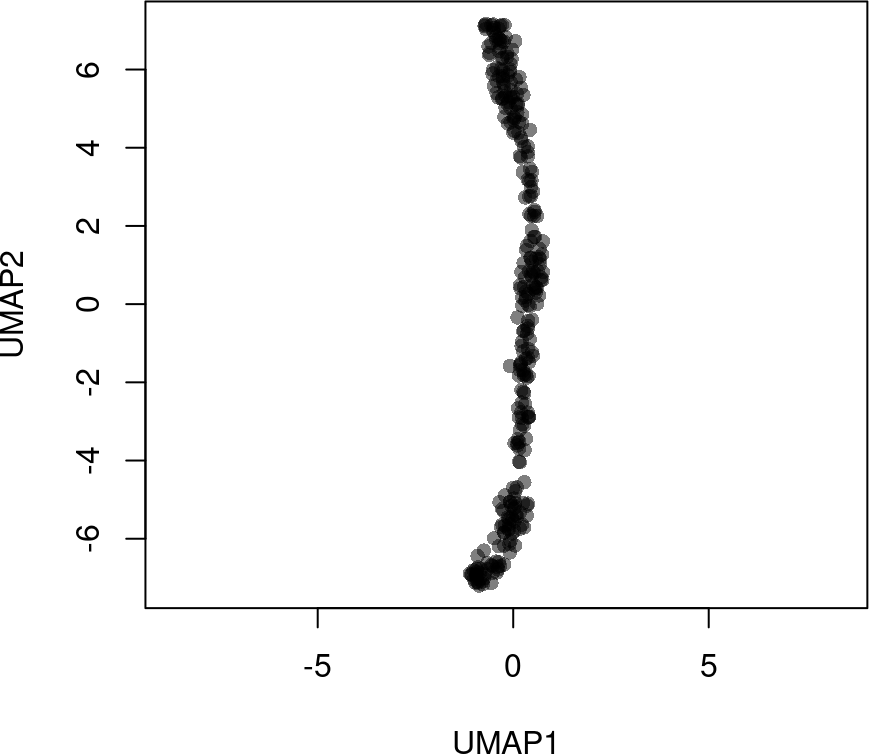We will add both dimensionality reductions to the SingleCellExperiment object, but continue our analysis focusing on the PCA results.

reducedDims(sce) <- SimpleList(PCA = rd1, UMAP = rd2)

## 2.4 Clustering Cells

The final input to slingshot is a vector of cluster labels for the cells. If this is not provided, slingshot will treat the data as a single cluster and fit a standard principal curve. However, we recommend clustering the cells even in datasets where only a single lineage is expected, as it allows for the potential discovery of novel branching events.

The clusters identified in this step will be used to determine the global structure of the underlying lineages (that is, their number, when they branch off from one another, and the approximate locations of those branching events). This is different than the typical goal of clustering single-cell data, which is to identify all biologically relevant cell types present in the dataset. For example, when determining global lineage structure, there is no need to distinguish between immature and mature neurons since both cell types will, presumably, fall along the same segment of a lineage.

For our analysis, we implement two clustering methods which similarly assume that Euclidean distance in a low-dimensional space reflect biological differences between cells: Gaussian mixture modeling and $$k$$-means. The former is implemented in the mclust package (Scrucca et al. 2016) and features an automated method for determining the number of clusters based on the Bayesian information criterion (BIC).

library(mclust, quietly = TRUE)
## Package 'mclust' version 6.0.0
## Type 'citation("mclust")' for citing this R package in publications.
##
## Attaching package: 'mclust'
## The following object is masked from 'package:mgcv':
##
##     mvn
cl1 <- Mclust(rd1)$classification colData(sce)$GMM <- cl1

library(RColorBrewer)
plot(rd1, col = brewer.pal(9,"Set1")[cl1], pch=16, asp = 1)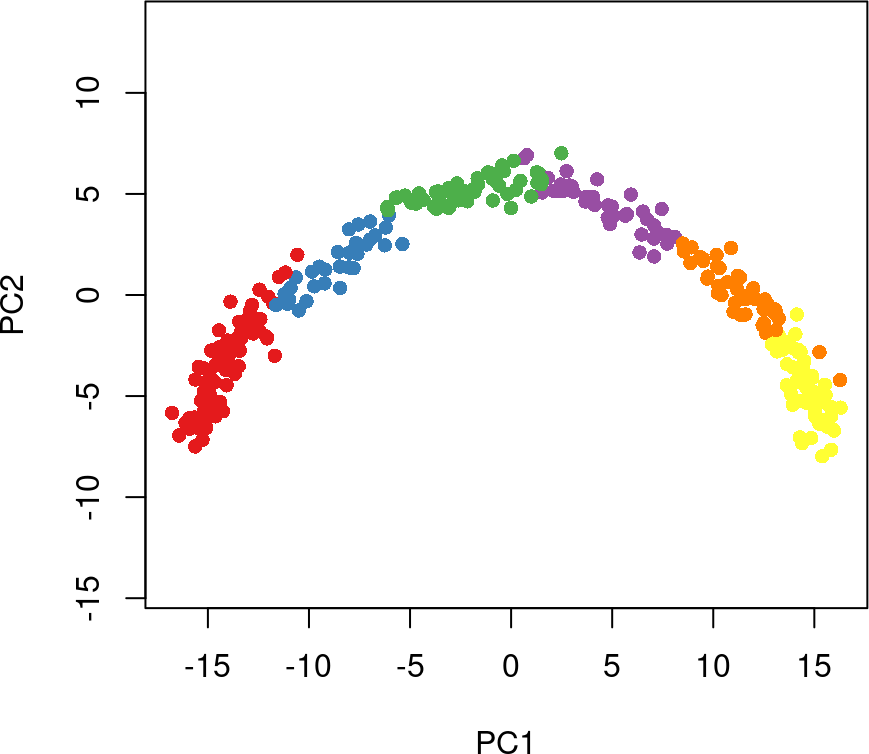While $$k$$-means does not have a similar functionality, we have shown in (Street et al. 2017) that simultaneous principal curves are quite robust to the choice of $$k$$, so we select a $$k$$ of 4 somewhat arbitrarily. If this is too low, we may miss a true branching event and if it is too high or there is an abundance of small clusters, we may begin to see spurious branching events.

cl2 <- kmeans(rd1, centers = 4)$cluster colData(sce)$kmeans <- cl2

plot(rd1, col = brewer.pal(9,"Set1")[cl2], pch=16, asp = 1)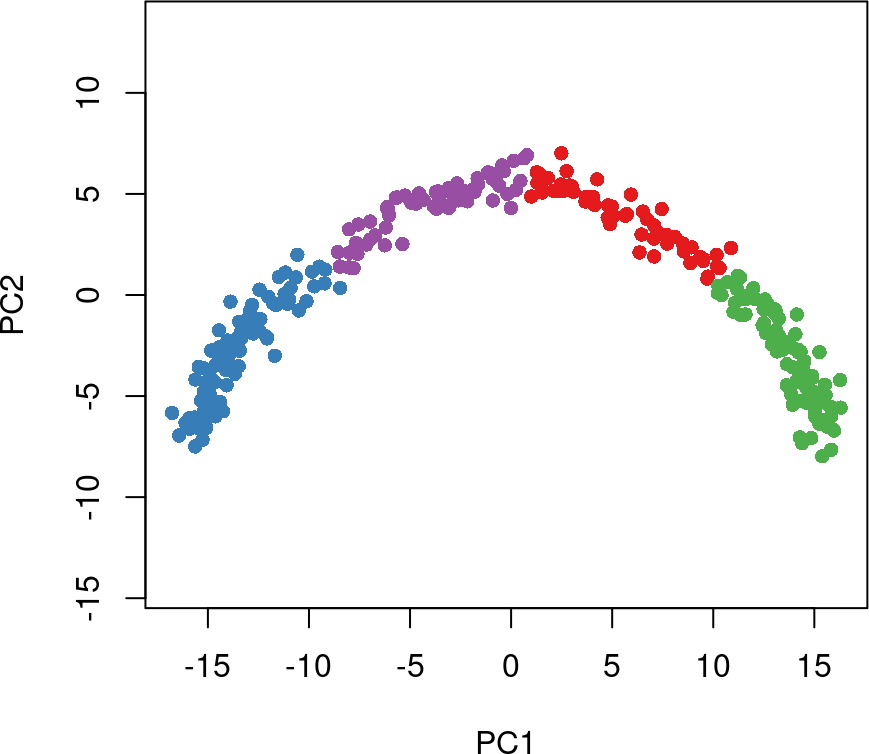# 3 Using Slingshot

At this point, we have everything we need to run slingshot on our simulated dataset. This is a two-step process composed of identifying the global lineage structure with a cluster-based minimum spanning tree (MST) and fitting simultaneous principal curves to describe each lineage.

These two steps can be run separately with the getLineages and getCurves functions, or together with the wrapper function, slingshot (recommended). We will use the wrapper function for the analysis of the single-trajectory dataset, but demonstrate the usage of the individual functions later, on the bifurcating dataset.

The slingshot wrapper function performs both steps of trajectory inference in a single call. The necessary inputs are a reduced dimensional matrix of coordinates and a set of cluster labels. These can be separate objects or, in the case of the single-trajectory data, elements contained in a SingleCellExperiment object.

To run slingshot with the dimensionality reduction produced by PCA and cluster labels identified by Gaussian mixutre modeling, we would do the following:

sce <- slingshot(sce, clusterLabels = 'GMM', reducedDim = 'PCA')

As noted above, if no clustering results are provided, it is assumed that all cells are part of the same cluster and a single curve will be constructed. If no dimensionality reduction is provided, slingshot will use the first element of the list returned by reducedDims.

The output is a SingleCellExperiment object with slingshot results incorporated. All of the results are stored in a PseudotimeOrdering object, which is added to the colData of the original object and can be accessed via colData(sce)$slingshot. Additionally, all inferred pseudotime variables (one per lineage) are added to the colData, individually. To extract all slingshot results in a single object, we can use either the as.PseudotimeOrdering or as.SlingshotDataSet functions, depending on the form in which we want it. PseudotimeOrdering objects are an extension of SummarizedExperiment objects, which are flexible containers that will be useful for most purposes. SlingshotDataSet objects are primarily used for visualization, as several plotting methods are included with the package. Below, we visuzalize the inferred lineage for the single-trajectory data with points colored by pseudotime. summary(sce$slingPseudotime_1)
##    Min. 1st Qu.  Median    Mean 3rd Qu.    Max.
##   0.000   8.631  21.121  21.414  34.363  43.185
library(grDevices)
colors <- colorRampPalette(brewer.pal(11,'Spectral')[-6])(100)
plotcol <- colors[cut(sce$slingPseudotime_1, breaks=100)] plot(reducedDims(sce)$PCA, col = plotcol, pch=16, asp = 1)
lines(SlingshotDataSet(sce), lwd=2, col='black')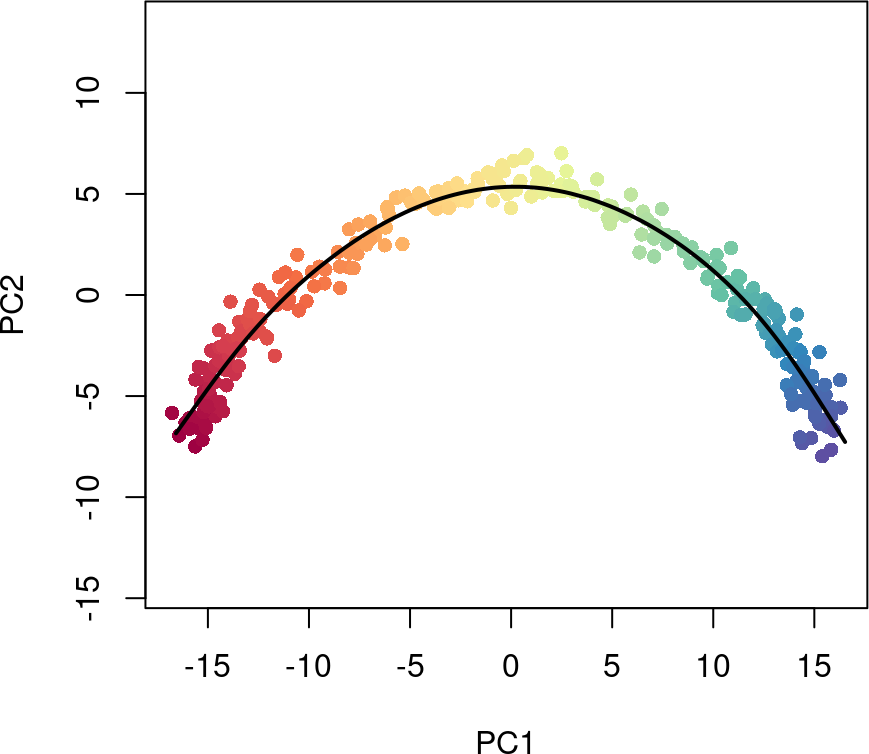We can also see how the lineage structure was intially estimated by the cluster-based minimum spanning tree by using the type argument.

plot(reducedDims(sce)$PCA, col = brewer.pal(9,'Set1')[sce$GMM], pch=16, asp = 1)
lines(SlingshotDataSet(sce), lwd=2, type = 'lineages', col = 'black')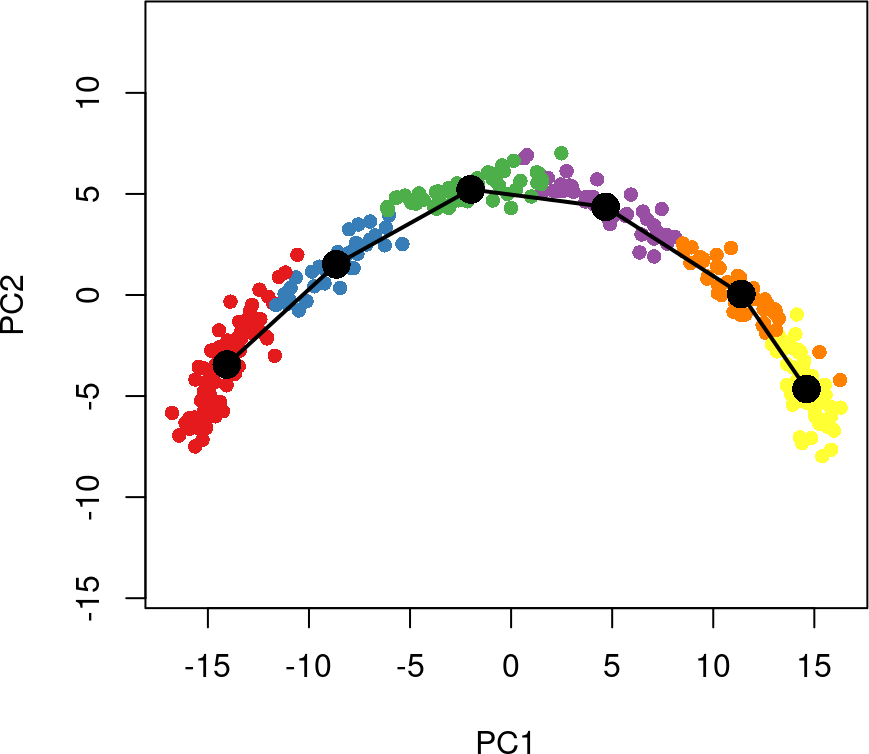# 4 Downstream Analysis

## 4.1 Identifying temporally dynamic genes

After running slingshot, we are often interested in finding genes that change their expression over the course of development. We will demonstrate this type of analysis using the tradeSeq package (Van den Berge et al. 2020).

For each gene, we will fit a general additive model (GAM) using a negative binomial noise distribution to model the (potentially nonlinear) relationshipships between gene expression and pseudotime. We will then test for significant associations between expression and pseudotime using the associationTest.

library(tradeSeq)

# fit negative binomial GAM
sce <- fitGAM(sce)

# test for dynamic expression
ATres <- associationTest(sce)

We can then pick out the top genes based on p-values and visualize their expression over developmental time with a heatmap. Here we use the top 250 most dynamically expressed genes.

topgenes <- rownames(ATres[order(ATres$pvalue), ])[1:250] pst.ord <- order(sce$slingPseudotime_1, na.last = NA)
heatdata <- assays(sce)$counts[topgenes, pst.ord] heatclus <- sce$GMM[pst.ord]

heatmap(log1p(heatdata), Colv = NA,
ColSideColors = brewer.pal(9,"Set1")[heatclus])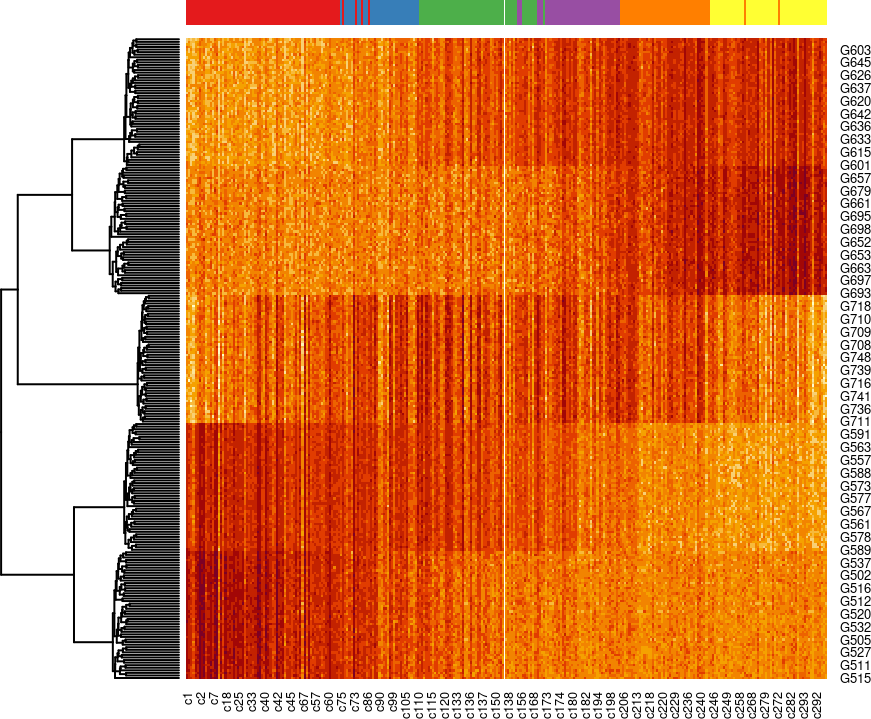# 5 Detailed Slingshot Functionality

Here, we provide further details and highlight some additional functionality of the slingshot package. We will use the included slingshotExample dataset for illustrative purposes. This dataset was designed to represent cells in a low dimensional space and comes with a set of cluster labels generated by $$k$$-means clustering. Rather than constructing a full SingleCellExperiment object, which requires gene-level data, we will use the low-dimensional matrix of coordinates directly and provide the cluster labels as an additional argument.

## 5.1 Identifying global lineage structure

The getLineages function takes as input an n $$\times$$ p matrix and a vector of clustering results of length n. It maps connections between adjacent clusters using a minimum spanning tree (MST) and identifies paths through these connections that represent lineages. The output of this function is a PseudotimeOrdering containing the inputs as well as the inferred MST (represented by an igraph object) and lineages (ordered vectors of cluster names).

This analysis can be performed in an entirely unsupervised manner or in a semi-supervised manner by specifying known initial and terminal point clusters. If we do not specify a starting point, slingshot selects one based on parsimony, maximizing the number of clusters shared between lineages before a split. If there are no splits or multiple clusters produce the same parsimony score, the starting cluster is chosen arbitrarily.

In the case of our simulated data, slingshot selects Cluster 1 as the starting cluster. However, we generally recommend the specification of an initial cluster based on prior knowledge (either time of sample collection or established gene markers). This specification will have no effect on how the MST is constructed, but it will impact how branching curves are constructed.

lin1 <- getLineages(rd, cl, start.clus = '1')
lin1
## class: PseudotimeOrdering
## dim: 140 2
## pathData names(0):
plot(rd, col = brewer.pal(9,"Set1")[cl], asp = 1, pch = 16)
lines(SlingshotDataSet(lin1), lwd = 3, col = 'black')